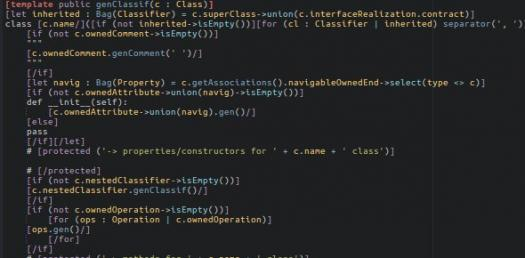# Python Programming Quiz 1

Approved & Edited by ProProfs Editorial Team
At ProProfs Quizzes, our dedicated in-house team of experts takes pride in their work. With a sharp eye for detail, they meticulously review each quiz. This ensures that every quiz, taken by over 100 million users, meets our standards of accuracy, clarity, and engagement.
| Written by Tomroy
T
Tomroy
Community Contributor
Quizzes Created: 1 | Total Attempts: 414
Questions: 11 | Attempts: 414SettingsEnd of first quarter test.

• 1.

### What is missing at the end of the following line? if x<=5

• A.

;

• B.

#

• C.

.

• D.

:

D. :
Explanation
A colon ":"

Rate this question:

• 2.

### Which is correct if the input I want is specifically a number?

• A.

• B.

• C.

• D.

Explanation
Although the word "year" doesn't correlate to age, the variable name can be anything that fits within the parameters of python.

Rate this question:

• 3.

### A1=random._______(1,10)

randint
Explanation
The correct answer is "randint". The function "randint" is used to generate a random integer between two specified numbers, in this case, between 1 and 10.

Rate this question:

• 4.

• 5.

### Which of the following are correct?

• A.

Print " Hello World "

• B.

Print = "Hello World"

• C.

Print " 'Hello World' "

• D.

Print ' Hello Word '

A. Print " Hello World "
C. Print " 'Hello World' "
D. Print ' Hello Word '
Explanation
The print = "Hello World" is not correct because "print" is a Python keyword and cannot be used as a variable name.

Rate this question:

• 6.

• 7.

• 8.

### Write a statement that picks a random number between 7 and 35 using the variable name "number"

number = random.randint(7,35)
Explanation
The given answer correctly assigns a random number between 7 and 35 to the variable named "number" using the random.randint() function. This function takes two arguments, the lower and upper bounds, and returns a random integer within that range. In this case, it will generate a random number between 7 and 35 (inclusive) and assign it to the variable "number".

Rate this question:

• 9.

### ______ = input("please enter a number")

anything
Explanation
Any variable name that is consistent with Python documentation.

Rate this question:

• 10.

### What line of code is necessary before executing a cammand picking a random number?

Import Random
Explanation
The line of code "Import Random" is necessary before executing a command to pick a random number because it imports the Random module in Python. The Random module provides functions that allow us to generate random numbers. By importing this module, we can access these functions and use them to pick a random number in our code.

Rate this question:

• 11.

### What is wrong with thr follong code?while guess = "F":

Related TopicsBack to top
×

Wait!
Here's an interesting quiz for you.